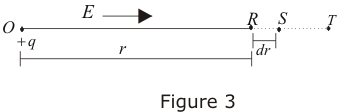# Relation between electric fields and Electric potential

## Relation between electric fields and electric potential

• Consider the electric field E due to a point charge +q at point O in a radially outward direction shown below in the figure.• Suppose R and S are two points at a distance r and r+dr from point O where dr is vanishingly small distance and V is electric potential at point R.
• Now force on any test charge q' at point R in terms of electric field is
F=q'E
• Work done by the force in displacing test charge from R to S in field of charge q is
dW = F·dr = q'E·dr
and, change in potential energy is
dU = -dW = -q'E·dr
Change in electric potential would be
dV = dU/q
or dV = -E·dr ----(11)
• From equation 11 electric field is
$E=- \frac {dV}{dr}$ ---(12)
The quantity $\frac {dV}{dr}$ is the rate of change of potential with the distance and is known as potential gradient. Negative sign in equation 12 indicates the decrease in electric potential in the direction of electric field.
• For Cartesian coordinate system
E=Exi+Eyj+Ezk
and,
dr=dxi+dyj+dzk
from equation 11
dV=-E . dr
or, $dV=-(E_x dx+E_y dy+E_zdz)$ ----(13)
• Thus components of E are related to corresponding derivatives of V in the following manner
$E_x=-\frac {dV}{dx}$ ---(14a)
$E_y=-\frac {dV}{dy}$ ---(14b)
$E_z=-\frac {dV}{dz}$ -----(14c)
In equation (14a) we see that V is differentiated with respect to coordinate x keeping other coordinates constant. Same is the case with equations (14b) and (14c) in case of y and z coordinates respectively.
• This can be written in partial derivative form as $E_x=-\frac{\partial V}{\partial x}$ ---(15a)
$E_y=-\frac{\partial V}{\partial y}$ ---(15b)
$E_z=-\frac{\partial V}{\partial z}$ -----(15c)
• So if we know Electric Field as function of position we can calculate V using Eq. 11, and if we know V as a function of position, we can calculate using Eq. 14 Deriving V from requires integration, and deriving from V requires differentiation.